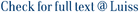This chapter starts with a description of the contractual features of CCTs and then points out the difformities compared with the theoretical prescriptions (Section 7 .2.1). The theoretical value of CCTs is then represented as the sum of both deterministic and uncertain future payments, discounted using tbe appropriate factors (Section 7.2.2). The next section describes the theoretical environment for the valuation of CCTs, with special reference to the floating rate coupons. After introducing the forward risk-adjusted process, defined by the Separation Theorem (Section 7.3.1), a simple generalisation of the formula for valuing an elementary floating rate coupon is presented (Section 7.3.2). Specifically, the formula permits the valuation of floating rate coupons when the base yield is forward and quoted for an interval different from the reference period (e.g. an annual yield which has to be transformed, under compound interest, into the semi-annual equivalent). A sensitivity analysis is then carried out to test the effects on the value of the floating rate coupons of changes in the state variable (the instantaneous interest rate), the parameters of the model and the contractual features of these elementary securities (Section 7.3.3). A test is then made of the accuracy of the forward approximation used in other studies. The third section first extends the formula for valuing elementary coupons to the case of CCTs linked to the gross yield on Italian T-bills. This is necessary when the pre-tax prices of T-bills are modelled (Section 7 . 4. 1). Besides, in order to obtain a valuation formula in the case of a link with average yields, portfolios consisting of several coupons with the same payment date are considered (Section 7.4.2). To permit the model to express below par theoretical values of CCTs, even with large spreads with respect to the reference parameter, an additional parameter has been added that captures the extra riskiness perceived by operators, especially as regards the future representativeness of the base yields (Section 7 .4.3). The chapter ends with a number of conclusions.

The valuation of Italian floating-rate Treasuries / Barone, Emilio; Folonari, F.. - (1994), pp. 127-164.

### The valuation of Italian floating-rate Treasuries

#### Abstract

This chapter starts with a description of the contractual features of CCTs and then points out the difformities compared with the theoretical prescriptions (Section 7 .2.1). The theoretical value of CCTs is then represented as the sum of both deterministic and uncertain future payments, discounted using tbe appropriate factors (Section 7.2.2). The next section describes the theoretical environment for the valuation of CCTs, with special reference to the floating rate coupons. After introducing the forward risk-adjusted process, defined by the Separation Theorem (Section 7.3.1), a simple generalisation of the formula for valuing an elementary floating rate coupon is presented (Section 7.3.2). Specifically, the formula permits the valuation of floating rate coupons when the base yield is forward and quoted for an interval different from the reference period (e.g. an annual yield which has to be transformed, under compound interest, into the semi-annual equivalent). A sensitivity analysis is then carried out to test the effects on the value of the floating rate coupons of changes in the state variable (the instantaneous interest rate), the parameters of the model and the contractual features of these elementary securities (Section 7.3.3). A test is then made of the accuracy of the forward approximation used in other studies. The third section first extends the formula for valuing elementary coupons to the case of CCTs linked to the gross yield on Italian T-bills. This is necessary when the pre-tax prices of T-bills are modelled (Section 7 . 4. 1). Besides, in order to obtain a valuation formula in the case of a link with average yields, portfolios consisting of several coupons with the same payment date are considered (Section 7.4.2). To permit the model to express below par theoretical values of CCTs, even with large spreads with respect to the reference parameter, an additional parameter has been added that captures the extra riskiness perceived by operators, especially as regards the future representativeness of the base yields (Section 7 .4.3). The chapter ends with a number of conclusions.
##### Scheda breve Scheda completa Scheda completa (DC)1994
0412597403
The valuation of Italian floating-rate Treasuries / Barone, Emilio; Folonari, F.. - (1994), pp. 127-164.
File in questo prodotto:
Non ci sono file associati a questo prodotto.
##### Pubblicazioni consigliate

I documenti in IRIS sono protetti da copyright e tutti i diritti sono riservati, salvo diversa indicazione.

Utilizza questo identificativo per citare o creare un link a questo documento: `https://hdl.handle.net/11385/168285`
##### Citazioni
•ND
•ND